Reach Us+44-1522-440391
Use of Non-Homogeneous Poisson Processes in the Modeling of Hospital over Admissions in Ribeirao Preto and Region, Brazil: An Application to Respiratory Diseases
Journal of Biometrics & Biostatistics

Like us on:

All submissions of the EM system will be redirected to Online Manuscript Submission System. Authors are requested to submit articles directly to Online Manuscript Submission System of respective journal.

Use of Non-Homogeneous Poisson Processes in the Modeling of Hospital over Admissions in Ribeirao Preto and Region, Brazil: An Application to Respiratory Diseases

Jorge Alberto Achcar1, Edilberto Cepeda-Cuervo2 and Edson Zangiacomi Martinez1*

1Universidade de Sao Paulo, FMRP Ribeirao Preto, SP, Brazil

*Corresponding Author:
Edson Zangiacomi Martinez
FMRP Ribeirao Preto, SP, Brazil
Email: [email protected]

Received Date: November 03, 2011; Accepted Date: November 29, 2011; Published Date: December 01, 2011

Citation: Achcar JA, Cepeda-Cuervo E, Martinez EZ (2012) Use of Non-Homogeneous Poisson Processes in the Modeling of Hospital over Admissions in Ribeirao Preto and Region, Brazil: An Application to Respiratory Diseases. J Biom Biostat 3:131. doi:10.4172/2155-6180.1000131

Copyright: © 2012 Achcar JA. This is an open-access article distributed under the terms of the Creative Commons Attribution License, which permits unrestricted use, distribution, and reproduction in any medium, provided the original author and source are credited.

Visit for more related articles at Journal of Biometrics & Biostatistics

Abstract

The daily number of hospital admission due to respiratory diseases can have a great variability. This variability could be explained by different factors as year seasons, temperature, pollution levels among many others [1,2]. In this paper, we have been using non-homogeneous Poisson processes with different intensity functions under the Bayesian paradigm and using standard existing MCMC (Markov Chain Monte Carlo) methods to simulate samples for the joint posterior distribution of interest. An application is given considering the daily number of hospital admission in Ribeirao Preto, Brazil in the period ranging from January 01, 1998 to December 31, 2007. The proposed model showed a good fit for the seasonality of the disease with simple interpretation in the framework of epidemiology.

Keywords

Hospital admissions; Respiratory diseases; Nonhomogeneous Poisson process; Bayesian inference; MCMC methods

Introduction

The daily counting of hospital admissions, has large variations due to several factors: year season, climatic variation, variation in levels of different pollutants, among many others. A great interest to public health administrators is related to the modeling of these daily counts, especially for cases of over admissions, which can result in many problems in the hospitals such as lack of beds and equipment, or lack of medicines and lack of health professionals. Counting of hospital admissions due to different causes has been modeled in different ways [3-7].

Among the various diseases that lead to hospitalizations, one stands out among all others: the respiratory diseases. According to data obtained in DATASUS (a Brazilian health data center), respiratory diseases, classified in Chapter X of the Tenth International Classification of Diseases (ICD-10) of the World Health organization (WHO), were the sixth leading cause of hospital admissions in Ribeirao Preto, in hospitals of the National Health System (SUS) in 2008.

The data for this study were provided by the Data Processing Center Hospital (PCHR, 2010) of the Department of Social Medicine, School of Medicine of Ribeirao Preto, University of Sao Paulo. We analyzed the period ranging from January 01, 1998 to December 31, 2007. This database was composed of variables characterizing the patient’s sex, age, occupation and town of residence, and variables characterizing the hospitalization as the time of entry and exit, exit condition, the main city of the diagnostic of the disease and where the patient was hospitalized.

A total of 25 municipalities were considered in this data set consisting of approximately 80,967 hospitalizations due to respiratory diseases with an average daily admissions equals to 22.4 and median equals to 22. We also observed that 75% of the observations had a value up to 28 admissions a day. Therefore we consider this point as the threshold for over admissions, that is, if there are 28 hospital admissions or more in a day, this will be considered an over admission. In figure 1 we observe the distribution of all daily admissions between January 01,1998 to November 31, 2007. In figure 2 we have the plot of the accumulated number of hospitalizations due respiratory diseases against time (days) when this occurs.

This paper has as main goal the counting modeling of hospital over admissions due to respiratory diseases using non-homogeneous Poisson processes with different intensity functions. Different intensity functions are considered and inferences for the proposed models are obtained under the Bayesian paradigm and using standard MCMC (Markov Chain Monte Carlo) methods.

The paper is organized as follows: in section 2 we introduce the modeling of hospital admissions; in section 3 the analysis of the respiratory data of Riberao Preto, and finally, in section 4, some conclusions.

Model and Methods

To model the number of times that hospital over admissions occur in Ribeirao Preto City, we consider a point process to count these violations. Let N = {Nt : t ∈ [0,T]} be the process that registers the cumulative number of daily over admissions that are observed during the interval (0,T), i.e., for each t ∈ [0,T], Nt is the number of hospital admission peaks that are observed during the time interval (0, t). Assume that N is modelled by a non-homogeneous Poisson process (NHPP) with intensity function,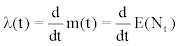(1)

where m(t) is the mean value function.

From the plot of Figure 1, we observe a cyclic and small decreasing behavior in the number of daily hospital admissions during the observed period. Therefore, as first modeling for the counting data, it is interesting to have an intensity function λ(t), t ≥ 0, that is a monotonic decreasing function of t.

Different parametrical forms for monotonic intensity functions are very popular within the framework of software reliability studies [8-10]. A popular form for the monotonic intensity functions is given by power law processes (PLP) or the Weibull intensity function.

Intensity functions assumed to model hospital over admissions

Let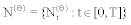be a non-homogeneous Poisson process with mean value function m(t|θ) where θ is a vector of parameters. The function m(t|θ) represents the expected number of events registered by N(Θ) up to time t (the hospital over admissions when the threshold is 28 admissions).

Equivalently, the process can be specified by its intensity function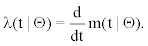(2)

In this paper we explore some especial cases of NHPP to analyse the hospital admissions data due to respiratory diseases of Ribeirao Preto: the power law (PLP); the Musa-Okumoto (MOP) ; the Goel- Okumoto (GOP)  and a generalized form of a Goel-Okumoto (GGOP) processes defined, respectively by the following mean value functions,

m(PLP) (t | θ) = (t | β)α, β, α > 0

m(MOP) (t | θ) = β log(1+t/α), β, α > 0

m(GOP) (t | θ) = α(1-exp(-βt)), β, α > 0

m(GGOP) (t | θ) = α(1-exp(-βtγ)), β, α, γ > 0                                               (3)

where θ =(α,β) for the PLP, MOP, and GOP models and θ = (α, β, γ) for the GGOP model. The intensity functions associated with those processes are given, respectively, by

λ(PLP) (t | θ) = (α/β)(t| β)α-1, β, α > 0

λ(MOP) (t | θ) = β/(t+α), β, α > 0

λ(GOP) (t | θ) = αβ exp(-βt)), β, α > 0

λ(GGOP) (t | θ) = αβγtγ-1(1-exp(-βtγ)), β, α, γ > 0                           (4)

We observe that:

1. The intensity function given by (2) defines the hazard rate of the time between occurrence of events in the respective models.

2. We observe from (4) that the intensity function λ(PLP)(t|Θ) gives a different behavior for the PLP depending on the value of α. We have that as a function of time that intensity function can be constant, decreasing or increasing depending on whether α = 1, α < 1 or α > 1 , respectively. The intensities λ(MOP)(t|Θ) and λ(GOP) (t|Θ) present a decreasing behavior as functions of t; and λ(GGOP) (t|Θ) describes the situation where the intensity increases slightly at the beginning and then begins to decrease with t.

The intensity functions given by (4) also were considered by Achcar et al. [22-24] to analyze ozone pollution data of Mexico City in presence or not of one or more change points.

Since we have a cyclic behavior for the hospital admission counting (Figure 1), we propose new intensity functions adding a cyclic term for the intensity functions (4). This additional term is given by

a(t) = δ cos(θt)                                                                              (5)

As a special case, a new MOP intensity function is given by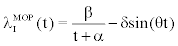(6)

The corresponding mean value function associated to the intensity function (6) is given by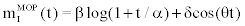(7)

In this model, δ is interpreted as the amplitude of oscillation and 1/θ as the period of the oscillation. In this model, the positivity of the λIMOP(t )needed to be guaranteed with appropriate selection of the prior distribution to have β/(T+α) > δθ. Other choices for the cyclic term can be made, as for example, with the sine function. These choices can be explored depending on the nature of the data set.

The likelihood function

Denoting the data set by DT = {n; t1, t2,…,tn; T} where n is the number of observed occurrence times which are such that 0 < t1 < t2 <… < tn < T, and where these values are the epochs of the occurrence of hospital over admissions up to time T, the likelihood function for θ considering the time truncated model is  given by,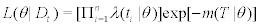(8)

We get inferences for the parameters of the model under a Bayesian approach. Bayesian inferences for NHPP has been discussed by many authors [12-14].

Posterior summaries of interest are obtained using standard MCMC (Markov Chain Monte Carlo) methods [15-17].

Analysis of the Hospital Over Admissions due to Respiratory Diseases

In this section, we analyze the daily hospital admissions count due to respiratory diseases in Riberao Preto region for the period ranging from January 01, 1998 to December 30, 2007. From this data set, we observe that in 948 days we have al least 28 hospital admissions due to respiratory diseases in the period of T = 3670 days.

To analyze this count data, we first assume a NHPP, considering the intensity functions (4) not in the presence of the cyclic term (5). For each proposed model, we assume the following prior distributions: α ~ U(0,2), β ~ U(0,100) for the PLP model; α ~ U(0,10000), β ~ U(0,3000) for the MOP model; α ~ U(1,10000), β ~ U(0,1), for the GOP model, and α ~ U(0,5000), β ~ U(0,0.001) and γ ~ U(0.5,1.5) for the GGOP model, where U(a,b) denotes an uniform distribution in the interval (a,b). We further assume prior independence among the parameters.

Observe that we are using approximately non-informative priors for the parameters of each model. Considering these priors, we obtained a proper joint posterior distribution of interest considering our data set (we have used standard uniform priors limited to a large finite interval) and in our case, we have an informative likelihood function. It is important to point out that identifiability issues and property of the posterior distribution of interest has been discussed by many authors, especially within the analysis of hierarchical models considering improper Jeffreys or Berger and Bernardo’s reference priors for the parameters of the model [18-20]. We also observed that with small modifications in the hyperparameter values of the prior distributions, we obtained similar posterior summaries of interest. In some cases, the choice of the hyperparameter values was made to have convergence of the Gibbs Sampling algorithm using the WinBugs software , possibly a numerical limitation of the software.

For each proposed model, we simulated 3000 initial Gibbs Samples considered as “burn-in-sample” to eliminate the effects of the initial values; after this “burn-in-sample” period we simulated another 30,000 Gibbs samples taking every 30th sample, to have approximately uncorrelated samples, which totalizes 1000 Gibbs samples.

In this simulation procedure, we have used the WinBugs software . Convergence of the Gibbs Sampler algorithm was monitored using standard existing methods as the traceplots of the simulated samples.

In Table 1, we have the posterior means, posterior standard deviations and 95% credible intervals for all parameters.

Model Parameter Mean S. D. 95% Credible interval
α 0.9232 0.03081 (0.867, 0.9848)
PLP β 2.244 0.5621 (1.311, 3.504)
MOP α 6964.0 1511.0 (4185.0,9657.0)
β 2238.0 399.1 (1505.0, 2921.0)
GOP α 2818.0 719.0 (1943.0, 4624.0)
β 0.0001205 0.0000298 (0.000064, 0.000182)
GGOP α 2229.0 655.6 (1502.0, 4248.0)
β 0.000104 0.000028 (0.000061, 0.00017)
γ 1.058 0.05368 (0.9554, 1.159)
MOP with α 4421.0 1071.0 (2862.0,7115.0)
cyclic factor β 1580.0 288.7 (1156.0, 2298.0)
δ 7.694 0.6225 (6.455, 8.840)
θ 0.01782 0.000031 (0.0178, 0.01789)

Tabel 1: Posterior sumaries.

In Figure 3 we have the plots of the empirical accumulated counts and the fitted mean value functions assuming each proposed model (panels a, b, c and d) versus time for occurrence of over admissions. From these plots, we observe that the MOP model gives better fit, but this could be improved considering the cyclic factor (6) in the MOP intensity function (intensity function 6).

Figure 3: Empirical accumulated counts and fitted mean value functions for (a) PLP, (b) MOP, (c) GOP and (d) GGOP models.

In this way, we assume as fifth model, the MOP model in presence of the cyclic factor (5) and assuming the following priors: α ~ U(2000,10000), β ~ U(1000,3000), δ ~ U(3,10), β ~ U(0.01,0.5). We also assume prior independence between the parameters. In this case, we have used some information of the previous Bayesian analysis not considering the presence of the cyclic factor (5) to choose the hyperparameter values for the prior distributions.

From the WinBugs output considering a “burn-in-sample” of size 5000 and 20000 simulated samples taking every 20th samples we also have in Table 1, the posterior summaries of interest for the MOP with intensity function (6). The convergence was monitored using traceplots of the Gibbs samples simulated for each parameter (Figure 4).

In Figure 5, we have the plots of the empirical accumulated counts and the fitted mean values assuming the MOP model in the presence of a cyclic factor (6) versus time of occurrence of over admissions. We observe a good fit of this model for the hospital over admissions counting due to respiratory diseases in Ribeirao Preto. In Figure 5, we also have 95% confidence bands for the fitted mean values.

In the MOP model, the intensity function is a positive decreasing function of time. In the proposed model the intensity is positive, but it is not decreasing as it was derived in the application. Figure 6 shows the standard behavior of the fitted intensity obtained in applications. We observe that the oscillation function decreases in each periodic point.

Conclussion

In this paper we introduced new modeling approaches to analyze count data due to hospital over admission. In our case, we considered hospital over admission due to respiratory diseases in Riberao Preto, Brazil, but this class of model could be applied to any disease. The use of non-homogeneous Poisson processes assuming different intensity functions gives a great flexibility of fit for the count data. We also introduced a new model considering the introduction of the cyclic term (6) to capture the seasonality of the disease. This new modeling approach could be used to analyze data from many different seasonal diseases.

The use of Bayesian methods considering standard existing MCMC simulation methods to generate samples for the joint posterior distribution of interest, especially using the WinBugs software gives a great simplification to get accurate inference results and accurate predictions.

These results could be of great interest in epidemiology and medical research.

References

Select your language of interest to view the total content in your interested language

Article Usage

• Total views: 12180
• [From(publication date):
January-2012 - Jun 26, 2019]
• Breakdown by view type
• HTML page views : 8359Can't read the image? click here to refresh